# c++ 基础——杂谈 2﻿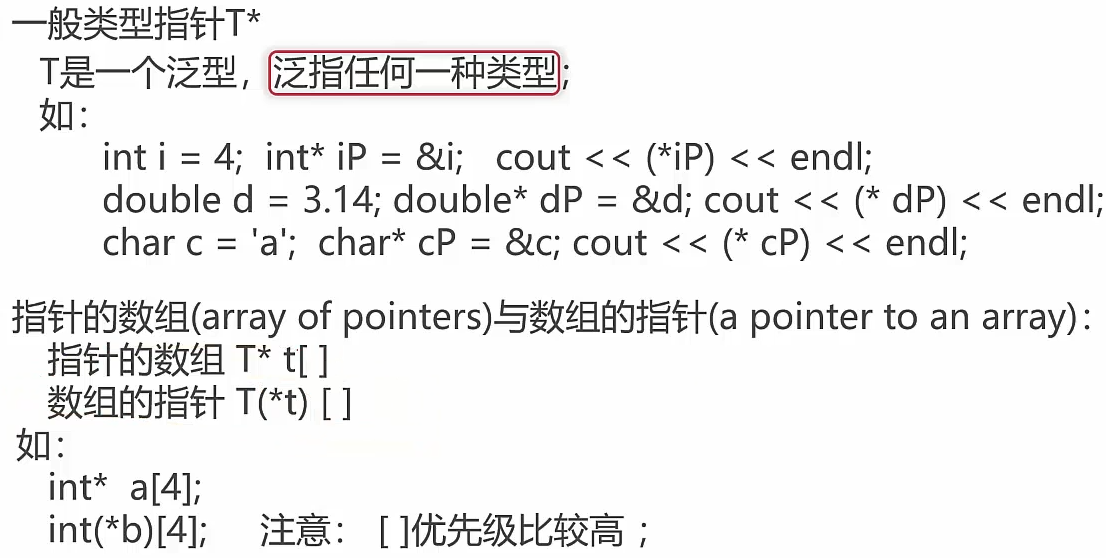int* a 指针的数组，4个都是存放int类型指针；

int(*b)数组的指针，指向的是int类型包含有5个元素的数组；

﻿

const pointer与pointer to const

    char strHelloworld[] = {"helloworld"};    char const *pStr1 = "helloworld";    char* const pStr2 = "helloworld";    char const * const pStr3 = "helloworld";

1. 看左侧最近的部分；

2. 如果左侧没有，就看右侧。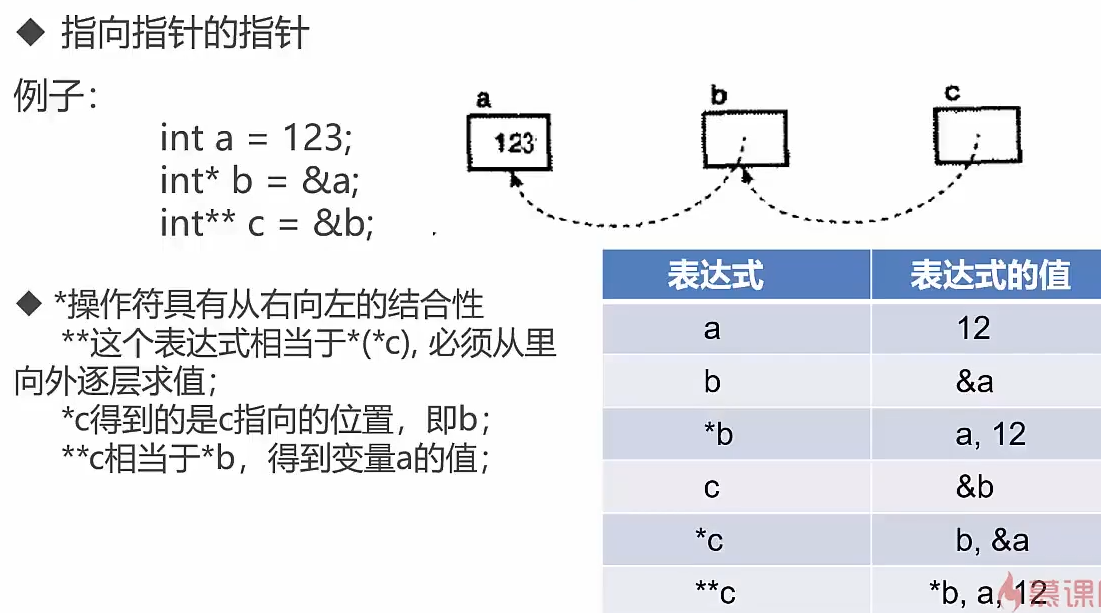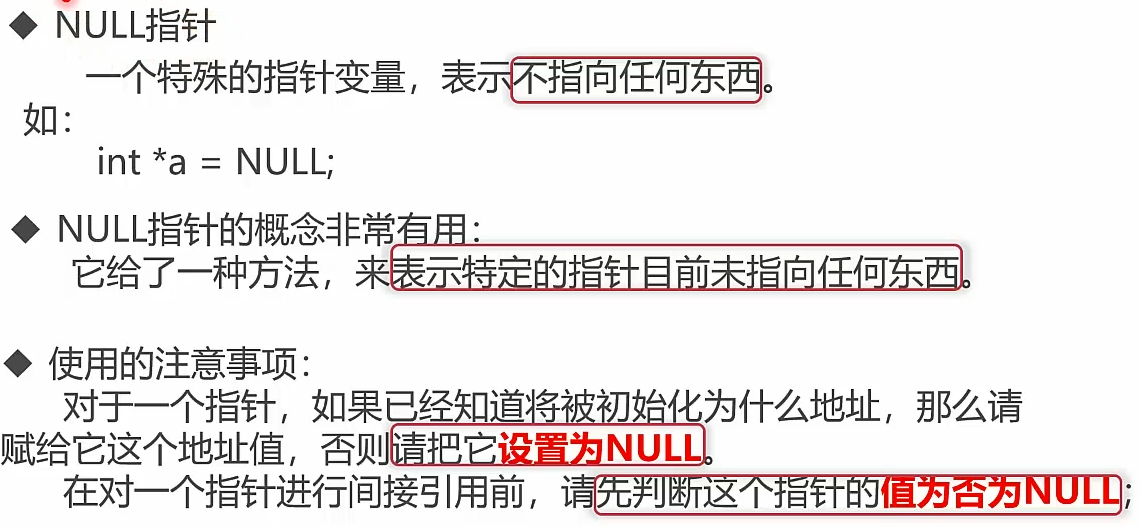int *pA = NULL;    pA = &a;    if (pA!=NULL){        cout<<(&pA)<<endl;    }    pA = NULL;

﻿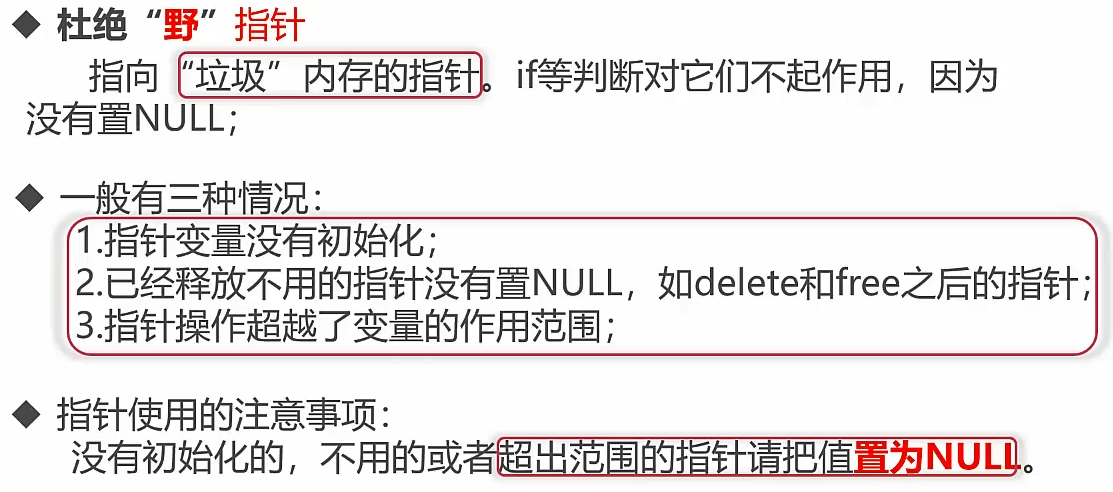﻿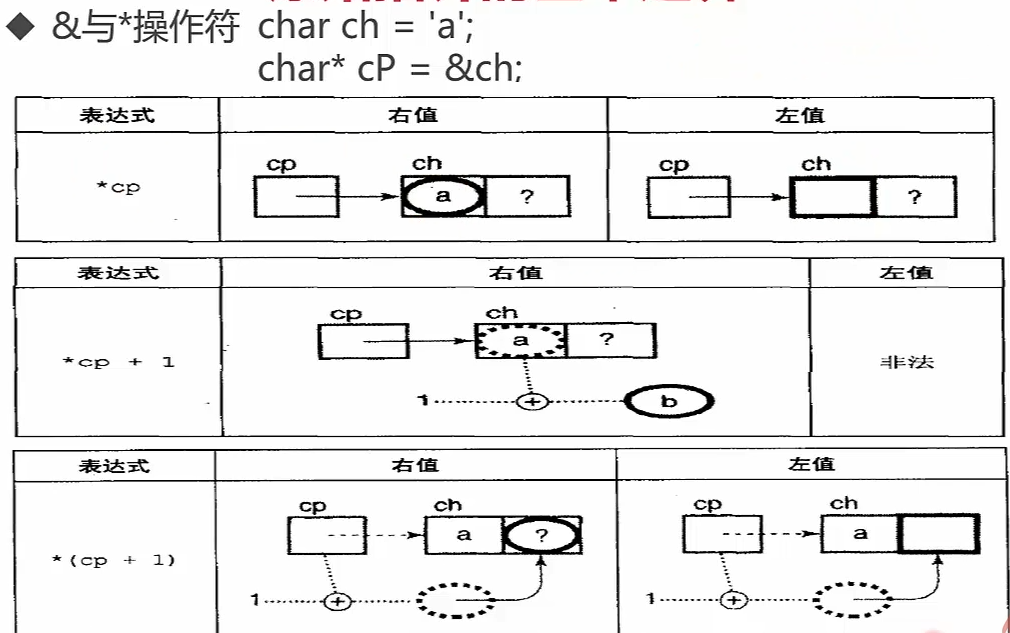﻿

char* cP = &ch;当char* cP = ‘b’；ch的值也会跟着改变。char ch2 = *ch;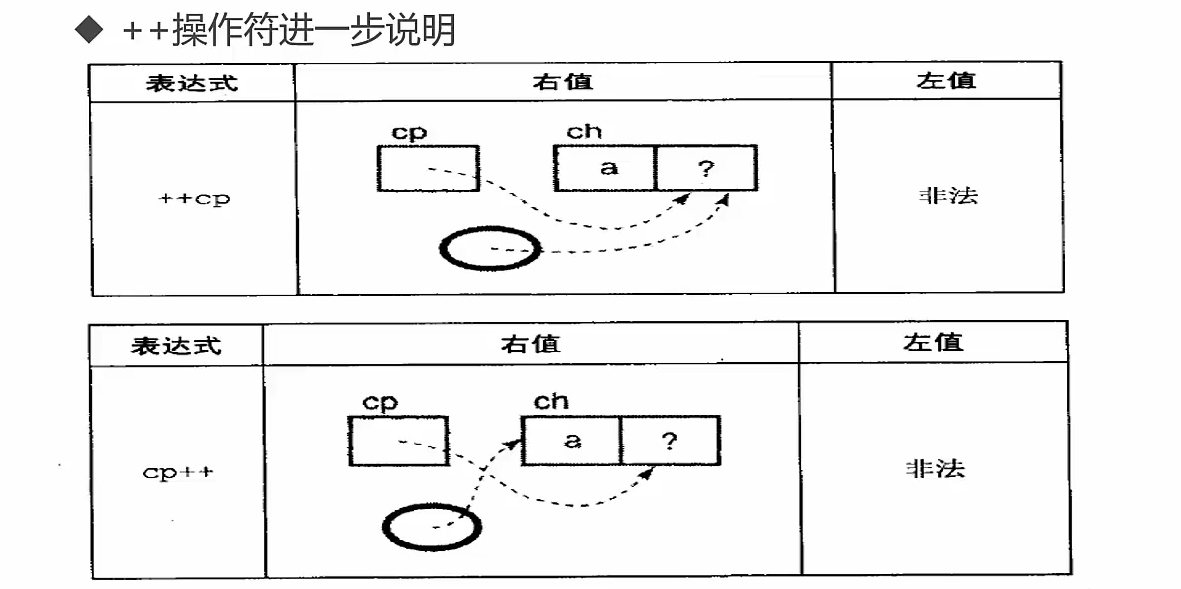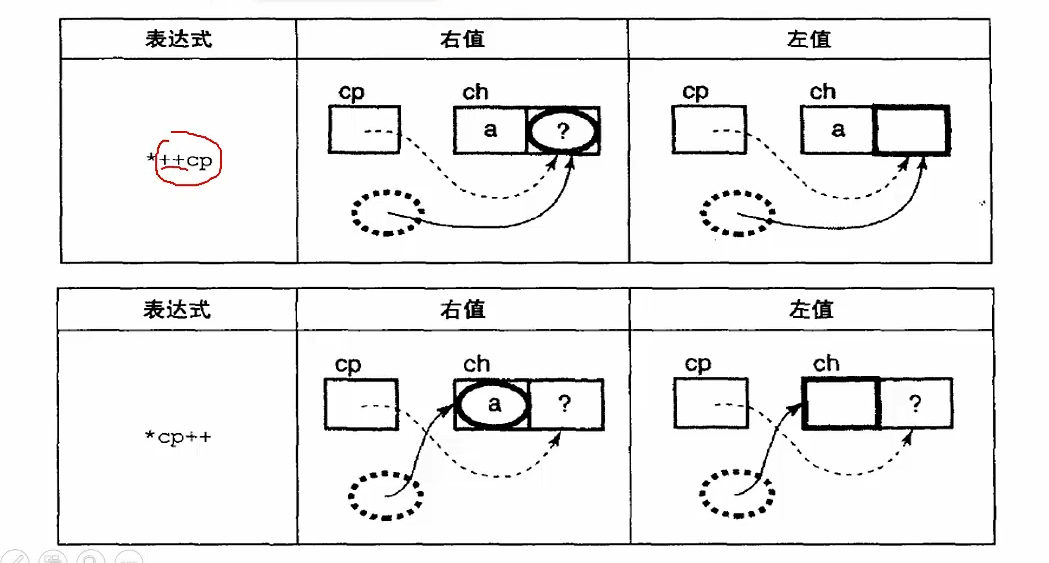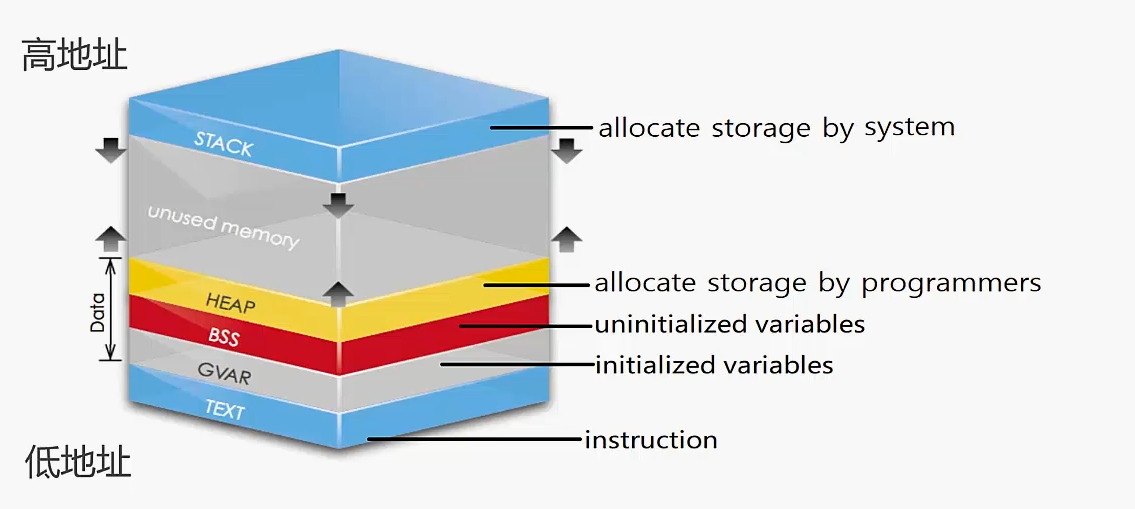BSS是没有初始化的变量

GVAR是已经初始化的变量（全局或者是静态的变量）

// demo_test2.cpp : 定义控制台应用程序的入口点。//#include "stdafx.h"int a = 0;  //全局初始化int *p1 ;  //全局未初始化int main(){	int b=1;   //栈区变量	char s[] = "abc";  //栈区变量	int *p2 = NULL;  //栈区变量	char *p3 = "123456";//p3在栈区，但是内部是在常量区域	static int c = 0;//全局区域	p1 = new int(10);//堆区域	p2 = new int(20);//堆区域  return 0;}

1 分配内存块

2 释放之前的内存块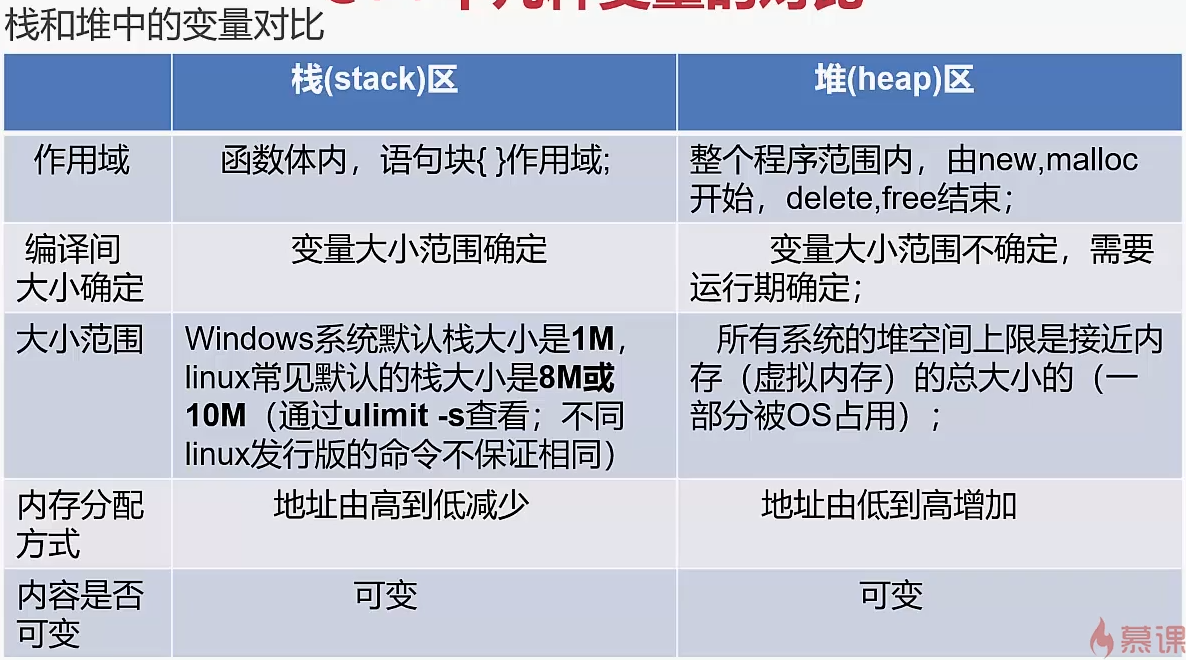﻿

c++资源管理方案--RAII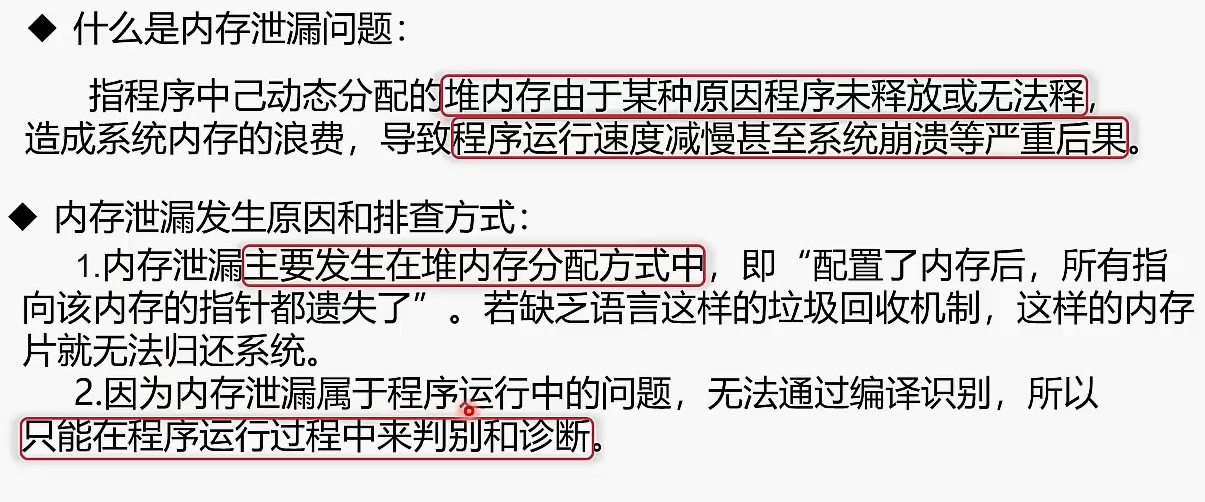﻿

unique_ptr , shared_ptr,weak_ptr，常用的几种智能指针

﻿

auto_ptr: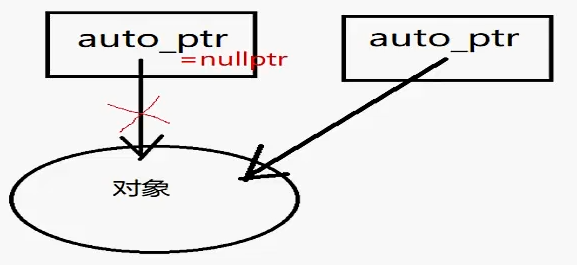﻿

// demo5-8.cpp : 定义控制台应用程序的入口点。//#include "stdafx.h"#include <string>#include <iostream>#include <memory>using namespace std;int main(){	{// 确定auto_ptr失效的范围		// 对int使用		auto_ptr<int> pI(new int(10));		cout << *pI << endl;                // 10 		// auto_ptr	C++ 17中移除	拥有严格对象所有权语义的智能指针		// auto_ptr原理：在拷贝 / 赋值过程中，直接剥夺原对象对内存的控制权，转交给新对象，		// 然后再将原对象指针置为nullptr（早期：NULL）。这种做法也叫管理权转移。		// 他的缺点不言而喻，当我们再次去访问原对象时，程序就会报错，所以auto_ptr可以说实现的不好，		// 很多企业在其库内也是要求不准使用auto_ptr。		auto_ptr<string> languages = {			auto_ptr<string>(new string("C")),			auto_ptr<string>(new string("Java")),			auto_ptr<string>(new string("C++")),			auto_ptr<string>(new string("Python")),			auto_ptr<string>(new string("Rust"))		};		cout << "There are some computer languages here first time: \n";		for (int i = 0; i < 5; ++i)		{			cout << *languages[i] << endl;		}		auto_ptr<string> pC;		pC = languages; // languges loses ownership. 将所有权从languges转让给pC，		//此时languges不再引用该字符串从而变成空指针		cout << "There are some computer languages here second time: \n";		for (int i = 0; i < 2; ++i)		{				cout << *languages[i] << endl;		}		cout << "The winner is " << *pC << endl;		//cout << "There are some computer languages here third time: \n";		//for (int i = 0; i < 5; ++i)		//{		//	cout << *languages[i] << endl;		//}	}	return 0; }

﻿

unique_ptr: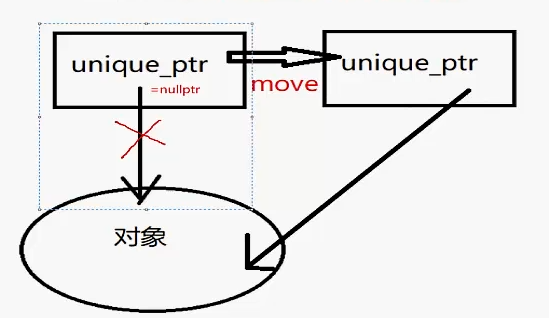﻿

// demo5-9.cpp : 定义控制台应用程序的入口点。//#include "stdafx.h"#include <memory>#include <iostream>using namespace std;int main(){	// 在这个范围之外，unique_ptr被释放	{		auto i = unique_ptr<int>(new int(10));		cout << *i << endl;	}	// unique_ptr	auto w = std::make_unique<int>(10);	cout << *(w.get()) << endl;                             // 10	//auto w2 = w; // 编译错误如果想要把 w 复制给 w2, 是不可以的。	//  因为复制从语义上来说，两个对象将共享同一块内存。	// unique_ptr 只支持移动语义, 即如下	auto w2 = std::move(w); // w2 获得内存所有权，w 此时等于 nullptr	cout << ((w.get() != nullptr) ? (*w.get()) : -1) << endl;       // -1	cout << ((w2.get() != nullptr) ? (*w2.get()) : -1) << endl;   // 10    return 0;}

auto自动检测，推导类型

﻿

shared_ptr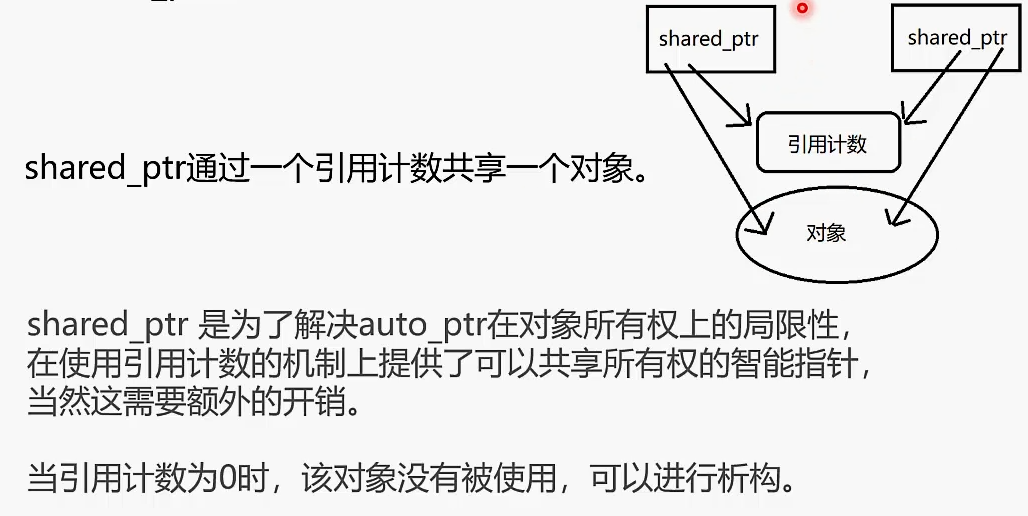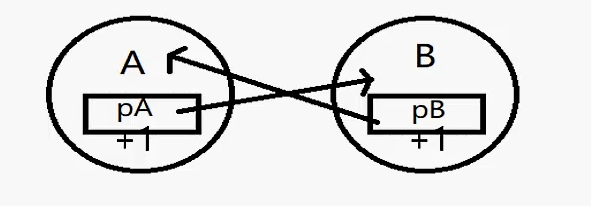﻿

weak_ptr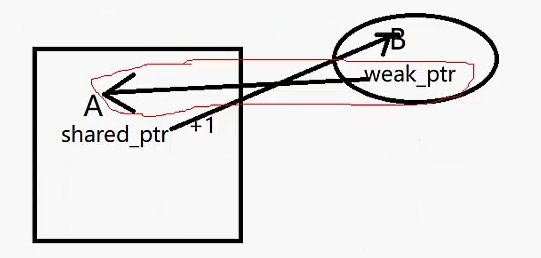A可以对B 造成直接的影响

B不能对A产生直接影响﻿

int x = 1;

int& rx = x;## 评论